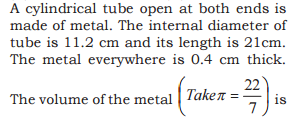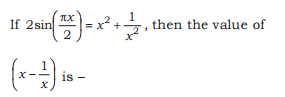# Maths Quiz for SSC CGL Tier-2

• #### 1.1. 5.375
2. 1
3. 6
4. 5
##### Answer And Explanation
Answer: Option C
Explanation:
Note:

# Maths Quiz for SSC CGL Tier-2

• #### 2.1. 316 cubic cm
2. 310 cubic cm
3. 306.24 cubic cm
4. 280.52 cubic cm
##### Answer And Explanation
Answer: Option C
Explanation:
Note:

# Maths Quiz for SSC CGL Tier-2

• #### 3.1.  3
2.  3 /2
3. 2+√ 3
4. 2-√ 3
##### Answer And Explanation
Answer: Option A
Explanation:
Note:

# Maths Quiz for SSC CGL Tier-2

• #### 4.The sides of a traingle are 3 cm, 4 cm and 5 cm. The area (in square cm) of the traingle formed by joining the mid points of the sides of the traingle is:

1. 6
2. 3
3. 3/2
4. 3/4
##### Answer And Explanation
Answer: Option C
Explanation:
Note:

# Maths Quiz for SSC CGL Tier-2

• #### 5.1. -1
2. 2
3. 1
4. 0
##### Answer And Explanation
Answer: Option D
Explanation:
Note:

# Maths Quiz for SSC CGL Tier-2

• #### 6.ABC is a right angled traingle where angle B = 90 degree and BD is perpendicular to AC. Which of the following relation is correct?

1. AB+ AD2 = 2 AC x 2 DC
2. AB2 = AC x AD
3. AB2 = AD x DC
4. AB2 - BD = BD x DC
##### Answer And Explanation
Answer: Option B
Explanation:
Note:AD/AB = AB/ AC

# Maths Quiz for SSC CGL Tier-2

• #### 7.1. 2 Cos2X
2. 2Cos2X
3. 2CosX
4. 2CosX/2
##### Answer And Explanation
Answer: Option C
Explanation:
Note:

# Maths Quiz for SSC CGL Tier-2

• #### 8. A man rides at the rate of 300 Metres per minute and stops for 6 minutes to change horses at the end of every sixth Kilometre. Then to travel a distance of 84 km, the time taken by the man is

1. 3 hours 15 minutes
2. 6 hours 17 minutes
3. 5 hours 18 minutes
4. 2 hours 17 minutes
##### Answer And Explanation
Answer: Option C
Explanation:
Note: Speed of horse rider = 350 metre/minute = 21 km/h

84/6 = 14 . It means, he will change the horse 13 times.

Time taken in changing the horses = 13 x 6 = 78 minutes
Time taken in covering 84 km = 4 hours
Total time = 4 hours + 78 minutes
= 5 hours 18 minutes

1. 1 km
2. 10 m
3. 10 cm
4. 1 m

# Maths Quiz for SSC CGL Tier-2

• #### 10. A vessel is filled with liquid 3 parts of which are water and 5 parts syrup. How many parts of mixture should be taken out and replaced by water so that container contains half milk and half water.

1. 1/3
2. 1/5
3. 2/5
4. 2/3
##### Answer And Explanation
Answer: Option B
Explanation:
Note:

#### Post a Comment

Leave your comment but never use bad words otherwise you will be blocked.